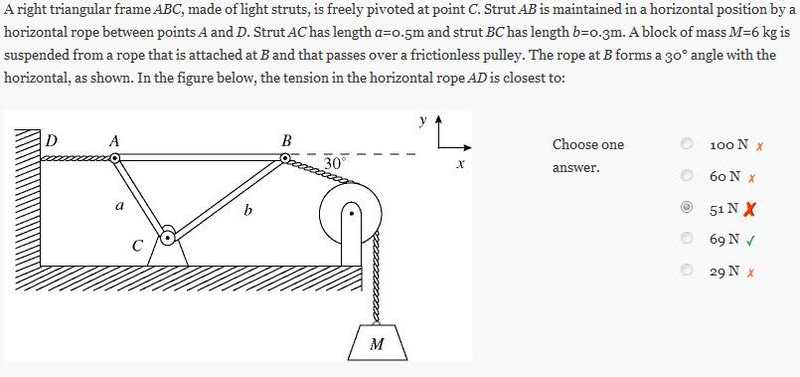# Tension in a pulley System

• Shambles

## Homework Statement## Homework Equations

F=mg=(6kg)(9.81m/s^2)=58.86N
Fx=(cos30)(58.86N)

## The Attempt at a Solution

Obviously the triangle somehow interferes with the tension between the top of the pulley and the rope attached to the wall. What really confuses me is how the tension in the rope attached to the wall is greater than the gravitational force of the weight itself. By my thinking the Fx at the top of the pulley = 50.97N which is wrong. How is the triangle increasing the tension?